Next: Simple Monte Carlo Simulation Up: Examples of Applications Previous: Momentum Resolution

## Impact Parameter Resolution

Impact parameter resolution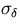is calculated as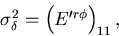(105)
where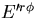is the full error matrix obtained from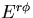given by Eq.3.2.48 by moving the pivot to the interaction point. In the pivot moving we can include the effect of thin-layer multiple scattering, for instance, at the beam pipe wall, according to the method explained in Section 2.4. If the material thickness is non-negligible, we need to use the method in Subsection 3.2.b instead. The effect of external coordinate information can also be implemented as explained there.

Let us consider again a tracking device having (n+1) equally sapced sampling points with an idential spatial resolution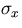.For simplicity, we further assume that multiple scattering in the tracking volume is negligible. Then the error matrix is given by Eq.B.7, where the pivot is located at y0 = 0 which is the -th hit position. When the -th hit is at r = Rin, we need to transform the error matrix by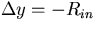, using Eq.3.2.19. Ignoring the material between the -th hit and the interaction point (by setting ES = 0), we obtain Fig.3.2 which plots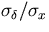as a function of the ratio of the extrapolation length and the lever arm length: Rin/(Rout-Rin).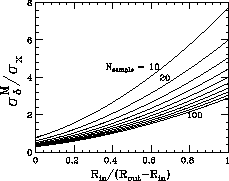Notice that the impact parameter resolution here is much worse in general than that expected from a straight-line approximation. This is because of the curvature error. If we have an external tracking device which provides additional curvature information, we can thus improve the impact parameter resolution significantly. We can do this by modifiying, according to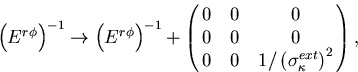(106)
and by following the same steps as above.Next: Simple Monte Carlo Simulation Up: Examples of Applications Previous: Momentum Resolution
Keisuke Fujii
12/4/1998